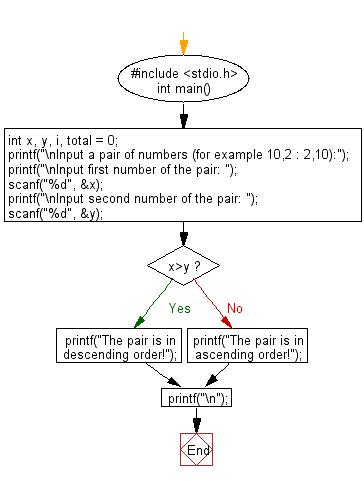﻿ C : Check 2 numbers in a pair is in ascending or descending

# C Exercises: Check whether two numbers in a pair is in ascending order or descending order

## C Basic Declarations and Expressions: Exercise-35 with Solution

Write a C program to check if two numbers in a pair are in ascending order or descending order.

C Code:

``````#include <stdio.h>
int main() {
int x, y, i, total = 0; // Declare variables for user input and calculations
printf("\nInput a pair of numbers (for example 10,2 : 2,10):");
printf("\nInput first number of the pair: ");
scanf("%d", &x); // Read the first number
printf("\nInput second number of the pair: ");
scanf("%d", &y); // Read the second number

if (x > y) {
printf("The pair is in descending order!"); // If x is greater than y, print message for descending order
} else {
printf("The pair is in ascending order!"); // Otherwise, print message for ascending order
}

printf("\n");
return 0;
}
``````

Sample Output:

```Input a pair of numbers (for example 10,2 : 2,10):
Input first number of the pair: 10

Input second number of the pair: 2
The pair is in descending order!
```

Flowchart:C Programming Code Editor:

What is the difficulty level of this exercise?

Test your Programming skills with w3resource's quiz.

﻿